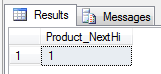# Using HiLo with FluentNHibernate

posted on 24 Oct 2010 | NHibernate

I spend ages trying to find some documentation or tutorial on how to use HiLo with FluentNHibernate after reading:

As it turns out, it's really easy to setup. I figured out two ways of doing it, the HiLo table having a single row, with each column for each table 'Hi'. Or a row for each table.

I setup a really simple example with the following table.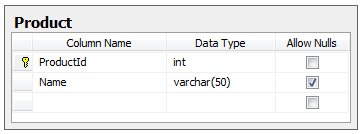'ProductId' is set to be int/primary key, but identity is false, since we don't want the database generating the id.

The two HiLo tables are like so:

### Row Per Table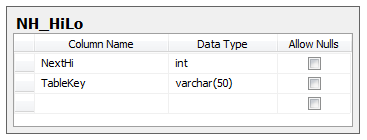### Column Per Table

(shows only a single column, but for each table you would add a new column)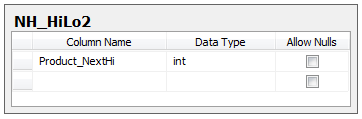Then all you need to do is setup the Fluent Mappings.

## Row Per Table

So for Row Per Table, the mapping for Product would look like this:

``````public class ProductMap : ClassMap<Product>
{
public ProductMap()
{
Id(x => x.Id).Column("ProductId")
.GeneratedBy
.HiLo("NH_HiLo", "NextHi", "1000", "TableKey = ''Product''");
Map(x => x.Name);
}
}
``````

HiLo takes the parameters (TableName, ColumnName, MaxLo, Where). Where is an override that we will use since we need to specify the row to return. So if we insert a single row into our table: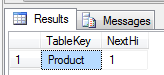We pass in our where clause as `TableKey = "Product"`, so that it knows which row to get the NextHi from.

For each new table we would just add a new row and a default NextHi value. Every time the SessionFactory is created it would grab the NextHi value and increment it by 1.

## Column Per Table

Column Per Table is very similar, except we don't need the where parameter, we only need to specify the column to look at. Assuming we were using the Column Per Table with the HiLo2 table, our mapping would look like this:

``````public class ProductMap : ClassMap<Product>
{
public ProductMap()
{
Id(x => x.Id).Column("ProductId")
.GeneratedBy
.HiLo("NH_HiLo2", "Product_NextHi", "1000");
Map(x => x.Name);
}
}
``````

We would need to add a single row to our table like so: